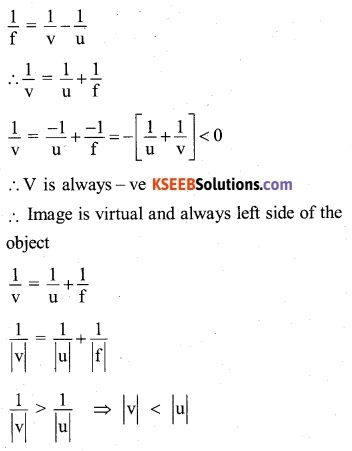# 2nd PUC Physics Question Bank Chapter 9 Ray Optics and Optical Instruments

## Karnataka 2nd PUC Physics Question Bank Chapter 9 Ray Optics and Optical Instruments

### 2nd PUC Physics Ray Optics and Optical Instruments NCERT Text Book Questions and Answers

Question 1.
A small candle, 2.5 cm in size is placed at 27 cm in front of a concave mirror of radius of curvature 36 cm. At what distance from ’ the mirror should a screen be placed in order to obtain a sharp image? Describe the nature and size of the image. If the candle is moved closer to the mirror, how would the screen have to be moved?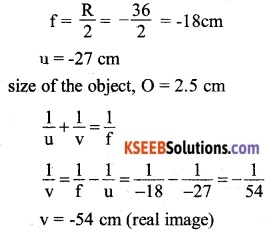Therefore, the screen should be placed at a distance of 54cm from the mirror on the same side as the object,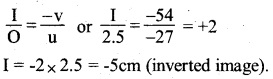When the candle is moved closer to the mirror, the image will move away from the mirror and likewise, the screen has to be moved away to bring the image on the screen. However, when the candle is at a distance less than 18cm, the image formed will be virtual. In that situation, the image cannot be brought to the screen.Question 2.
A 4.5 cm needle is placed 12 cm away from a convex mirror of focal length 15 cm. Give the location of the image and the magnification. Describe what happens as the needle is moved farther from the mirror.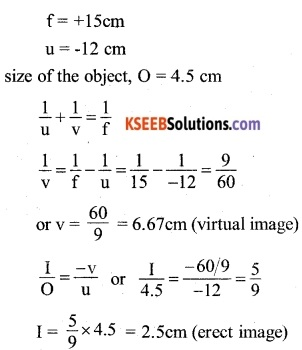When the needle is moved farther from the mirror, the image also moves away from the mirror but will not go beyond the focus of the mirror.

Question 3.
A tank is filled with water to a height of 12.5 cm. The apparent depth of a needle lying at the bottom of the tank is measured by a microscope to be 9.4 cm. What is the refractive index of water? If water is replaced by a liquid of refractive index 1.63 up to the same height, by what distance would the microscope have to be moved to focus on the needle again?
When tank is filled with water :
Real depth = 12.5cm
Apparent depth = 9.4cm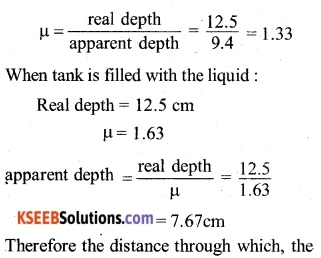Therefore objective and eyepiece should be placed at 11,67cm apart and object at a distance of 1,5cm from the objective.

Question 4.
A small telescope has an objective lens of focal length 140cm and an eyepiece of focal length 5.0cm. What is the magnifying power of the telescope for viewing distant objects when
(a) the telescope is in normal adjustment (i.e., when the final image is at infinity)?
(b) the final image is formed at the least distance of distinct vision (25cm)?
Here, f0 = 140cm, fe = 5.0cm
(a) When the telescope is in normal adjustment,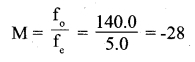(b) When the image is formed at least distance of distinct vision, then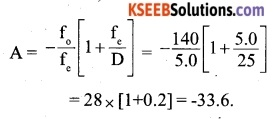Question 5.
(a) For the telescope described in Exercise 9.34 (a), what is the separation between the objective lens and the eyepiece?
(b) If this telescope is used to view a 100 m tall tower 3 km away, what is the height of the image of the tower formed by the objective lens?
(c) What is the height of the final image of the tower if it is formed at 25cm?
Here, f0 = 140cm; fe = 5.0cm
(a) The separation between the objective and the eye-piece,
L = f + f = 140 + 5 = 145 cm
(b) Let a be the angle formed by the object at the objective lens. Then,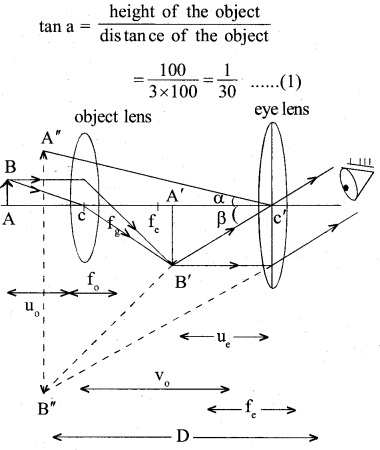Let A’ B’ be the height of the image produced by the objective lens at its focus. From the figure, it follows that the angle formed by the image a’ g’ at the objective lens is also equal to a.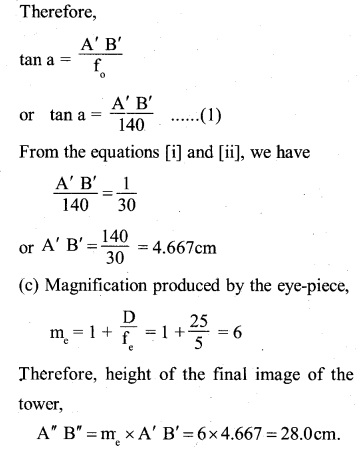Question 6.
A Cassegrain telescope uses two mirrors as shown in Fig. 9.33. Such a telescope is built with the mirrors 20mm apart. If the radius of curvature of the large mirror is 220mm and the small mirror is 140mm, where will the final image of an object at infinity be?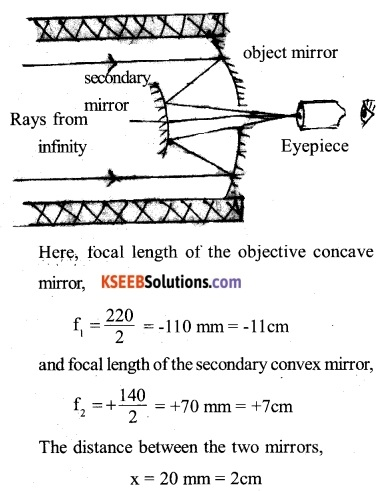When the object lies at infinity, the concave mirror will form the image of the object at its focus. As this image lies behind the convex mirror, it acts as a virtual object for the convex mirror.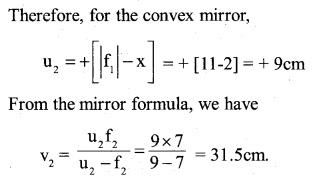Question 7.
Light incident normally on a plane mirror attached to a galvanometer coil retraces backwards as shown in Fig. 9.36. A current in the coil produces a deflection of 3.5o of the mirror. What is the displacement of the reflected spot of light on a screen placed 1.5 m away?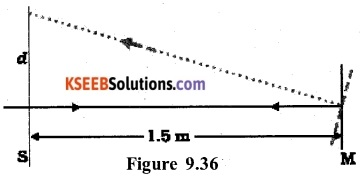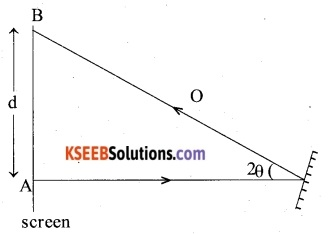When a plane mirror rotates through certain angle, the reflected ray turns through twice the angle of rotation. Therefore, angle between the incident ray AO and the reflected ray is,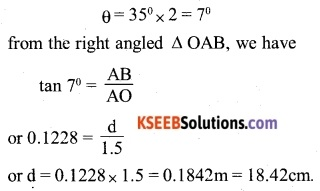Question 8.
Figure 9.37 shows an equiconvex lens (of refractive index 1.50) in contact with a liquid layer on top of a plane mirror. A small needle with its tip on the principal axis is moved along the axis until its inverted image is found at the position of the needle. The distance of the needle from the lens is measured to be 45.0cm. The liquid is removed and the experiment is repeated. The new distance is measured to be 30.0cm. What is the refractive index of the liquid?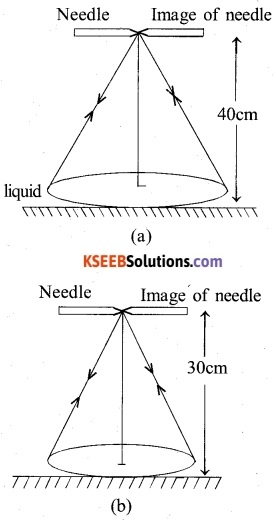The liquid between the convex lens and the plane mirror behaves as a plano-concave lens. Let f1 and f2 be the focal length of the convex lens and the liquid plano-concave lens. In the arrangement as shown in. Figure (b) as the needle and its image coincide with each other, the rays of light retrace their path. Therefore, the rays of light after refraction through the lens are falling normally on the plane mirror. It can happen so, if the needle lies at the focus of the convex lens. Hence, f, = 30cm From the lens maker’s formula, we have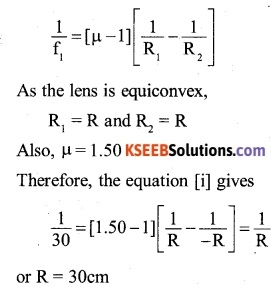In the arrangement as shown in Figure (a). As discussed above, now the needle lies at the focus of the combination of the two lenses. If F is the focal length of the combination of the two lenses, then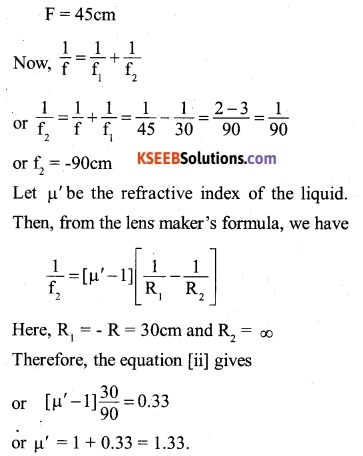### 2nd PUC Physics Ray Optics and Optical Instruments Additional Questions and Answers

Question 1.
Why is that sun-glasses (gaggles), which has curved surface, do not have any power?
The two surfaces of the goggle lens are parallel i.e. possess same radii of curvature. Since one surface is convex and other equally concave, the powers of the two surface is equal but of opposite signs. The total power of the goggle lens (p’) is the algebraic sum of the powers of the individual surfaces
p’ =p1 +p2 =p+(-p) = 0Question 2.
An illuminated object and a screen are placed 90 cm apart. Determine the focal length and nature of the lens required to produce a clear image on the screen, twice the size of the object.
Since the image is formed on the screen, it is a real image. Let the distance of the object from the lens be x. Then, distance of the image from the lens be (90-x)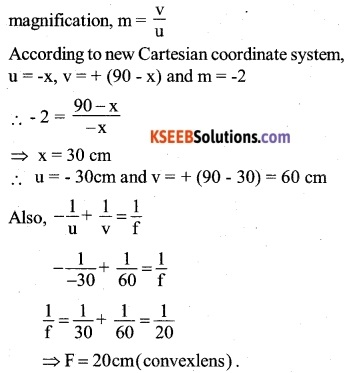Question 3.
Two lenses of power + 15D and -5D are in contact with each other forming a combination lens,
(a) what is the focal length of this combination?
(b) An object of size 3cm is placed at 30cm from this combination of lens. Calculate the position and size of the image formed.
(a) Power of the combination,
P = P1 + P2= 15 +(-5)= 10D
Therefore, focal length of the combination,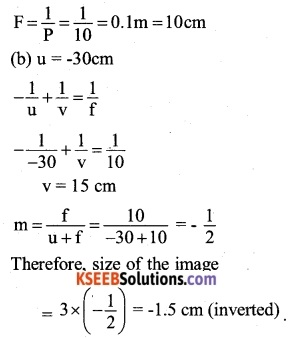Question 4.
A glass prism has a minimum angle of deviation 5 in air. State with reason, how the angle of minimum deviation will change, if the prism is immersed in a liquid of refractive index greater than 1.
We know that for a glass prism placed in air,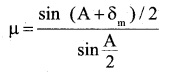when prism is immersed in a liquid of refractive index greater than 1, its refractive index w.r.t liquid will become less than p. From the above relation, it follows that the angle of minimum deviation will decrease.

Question 5.
Which of the following is used in optical fibres?
(A) Total internal reflection
(B) Scattering
(C) Diffraction
(D) Refraction
(A) Total internal reflection

Question 6.
An astronomical telescope has a large aperture to:
(A) Reduce spherical aberration
(B) Have high resolution
(C) Increase span of observation
(D) Have low dispersion.
(B) Have high resolution
The resolving power of the telescope is given by
$$R.P=\frac { D }{ 1.22\lambda }$$
∴ Resolving power of the telescope will be high, it its objective is of large aperture.Question 7.
The refractive index of the material of an equilateral prism is $$\sqrt { 3 }$$. What is the angle of minimum deviation?
(A) 45°
(B) 60″
(C) 37°
(D) 30°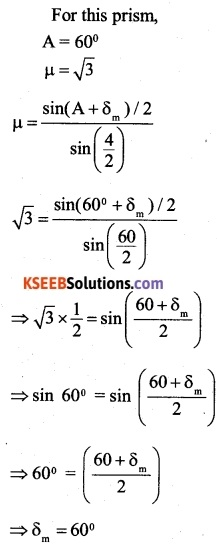Question 8.
Green light of wavelength 5460 is incident on an air-glass interface. If the refractive index of glass is 1.5, the wavelength of light in glass would be
(A) 3,640 Å
(B) 5460 Å
(C) 4861 Å
(D) None of the above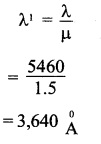Question 9.
A light wave of frequency V and wavelength X travels from air to glass. Then,
(A) V changes
(B) x does not change
(C) V does not change, X changes
(D) V and X changes
(C) V does not change, X changes

Question 10.
Two mirrors are kept at 60° to each other and a body is placed at middle. Total number of images formed is
(A) six
(B) four
(C) five
(D) three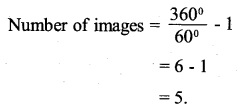(C) five

Question 11.
Two thin lenses of power +5D and -3D are in contact. What is the focal length of the combination (CBSE 2001)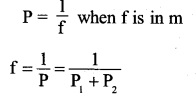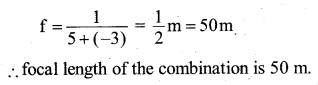Question 12.
A glass lens of refractive index 1.5 is kept in a liquid. What must be the refractive index of the liquid so that the lens will disappear  (CBSE 2008)
To disappear the lens the refractive index of liquid should be same i.e. 1.5.Question 13.
For which wave length is eye the most sensitive.
5500 A

Question 14.
Use mirror formula to show that for an object lying between the pole and the focus of a conceive mirror, the image formed is always virtual for a concave mirror (CBSE 2008)
f is negative and u is negative
Since object lying between the pole and focus u < f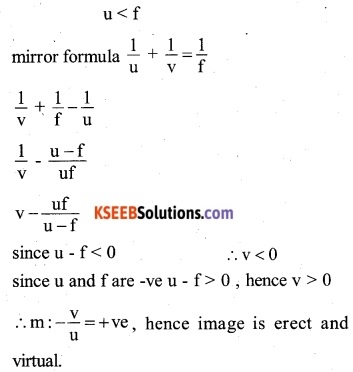Question 15.
Using Lens formula, show that a concave Lens produces a virtual and diminished image independent of location of object. (KU – 2009)